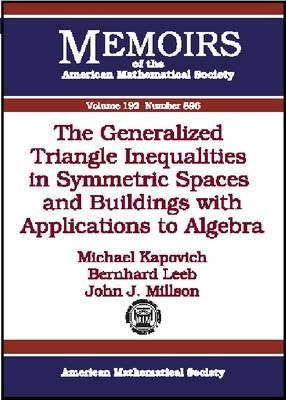Home » The Generalized Triangle Inequalities In Symmetric Spaces And Buildings With Applications To Algebra by Michael Kapovich# The Generalized Triangle Inequalities In Symmetric Spaces And Buildings With Applications To Algebra

## Michael Kapovich

Published April 1st 2008
ISBN : 9780821840542
Paperback
83 pages
Book Rating:Enter the sum

 About the Book In this paper the authors apply their results on the geometry of polygons in infinitesimal symmetric spaces and symmetric spaces and buildings to four problems in algebraic group theory. Two of these problems are generalizations of the problems ofMoreIn this paper the authors apply their results on the geometry of polygons in infinitesimal symmetric spaces and symmetric spaces and buildings to four problems in algebraic group theory. Two of these problems are generalizations of the problems of finding the constraints on the eigenvalues (resp. singular values) of a sum (resp. product) when the eigenvalues (singular values) of each summand (factor) are fixed. The other two problems are related to the nonvanishing of the structure constants of the (spherical) Hecke and representation rings associated with a split reductive algebraic group over \$/mathbb{Q}\$ and its complex Langlands dual. The authors give a new proof of the Saturation Conjecture for \$GL(/ell)\$ as a consequence of their solution of the corresponding saturation problem for the Hecke structure constants for all split reductive algebraic groups over \$/mathbb{Q}\$.